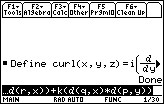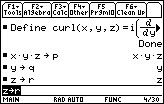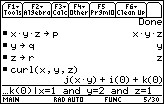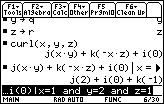# Knowledge Base

## Solution 12054: Finding the Curl of a Vector Field on the TI-89 Family, TI-92 Family, and Voyage™ 200 Graphing Calculators.

### How do I find the curl of a vector field on the TI-89 family, TI-92 family, and Voyage 200 graphing calculators?

The TI-89 family, TI-92 family, and Voyage 200 graphing calculators do not have a built in way to find the curl of a vector field. However, there is a work around to define it. To find the curl of the vector field refer to the example below.

Example: Find the curl of the vector field F.

F(x,y,z) = x * y * zi + yj + zk at point (1,2,1)

Solution:

1) Press [HOME] [F4] .
2) Press [2ND] [ALPHA] [c] [u] [r] [l] [ALPHA].
3) Press [(] [X] [,] [Y] [,] [Z] [)] [=].
4) Press [ALPHA] [i] [F3]  [(] [ALPHA] [r] [,] [Y] [)] [-].
5) Press [F3]  [(] [ALPHA] [q] [,] [Z] [)] [)] [+].
6) Press [ALPHA] [j] [F3]  [(] [ALPHA] [p] [,] [Z] [)] [-].
7) Press [F3]  [(] [ALPHA] [r] [,] [X] [)] [)] [+].
8) Press [ALPHA] [k] [F3]  [(] [ALPHA] [q] [,] [X] [)] [-].
9) Press [F3]  [(] [ALPHA] [p] [,] [Y] [)] [)].
10) Press [ENTER].

Please Note: Users with the TI-92 family or Voyage 200 can skip pressing [ALPHA].11) Press [X] [x] [Y] [x] [Z] [STO►] [ALPHA] [p] [ENTER].
12) Press [Y] [STO►] [ALPHA] [q] [ENTER].
13) Press [Z] [STO►] [ALPHA] [r] [ENTER].Please Note: X*Y*Z is just an example expression. Feel free to use whatever expressions describe the vector field in question.

14) Press [2ND] [ALPHA] [c] [u] [r] [l] [ALPHA].
15) Press [(] [X] [,] [Y] [,] [Z] [)] [ENTER].16) Press [▲] [ENTER] [|] [X] [=] .
17) Press [2nd]    [Y] [=] .
18) Press [2nd]    [Z] [=] .
19) Press [ENTER].Please see the TI-89 family, TI-92 family and Voyage 200 guidebooks for additional information.